High School Math : Understanding Derivatives of Exponents

Example Questions

Example Question #1 : Understanding The Derivative Of Exponents

Find the derivative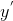for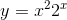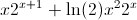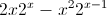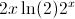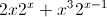Explanation:

The derivative must be computed using the product rule.  Because the derivative ofbrings adown as a coefficient, it can be combined with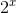to give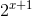Example Question #3 : Understanding The Derivative Of Exponents

Give the instantaneous rate of change of the function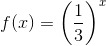at.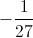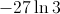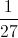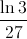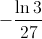Explanation:

The instantaneous rate of change ofat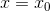is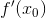, so we will find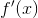and evaluate it at.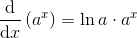for any positive, so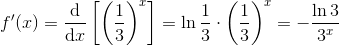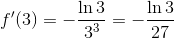Example Question #2 : Understanding The Derivative Of Exponents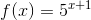What is?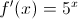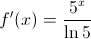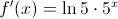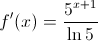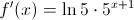Explanation: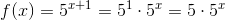Therefore,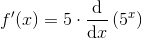for any real, so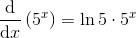, and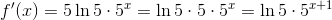Example Question #74 : Calculus I — Derivatives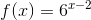What is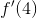?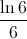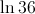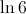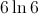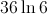Explanation: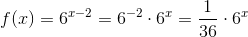Therefore,for any positive, so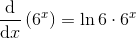, and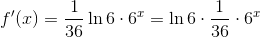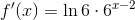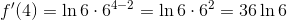All High School Math Resources